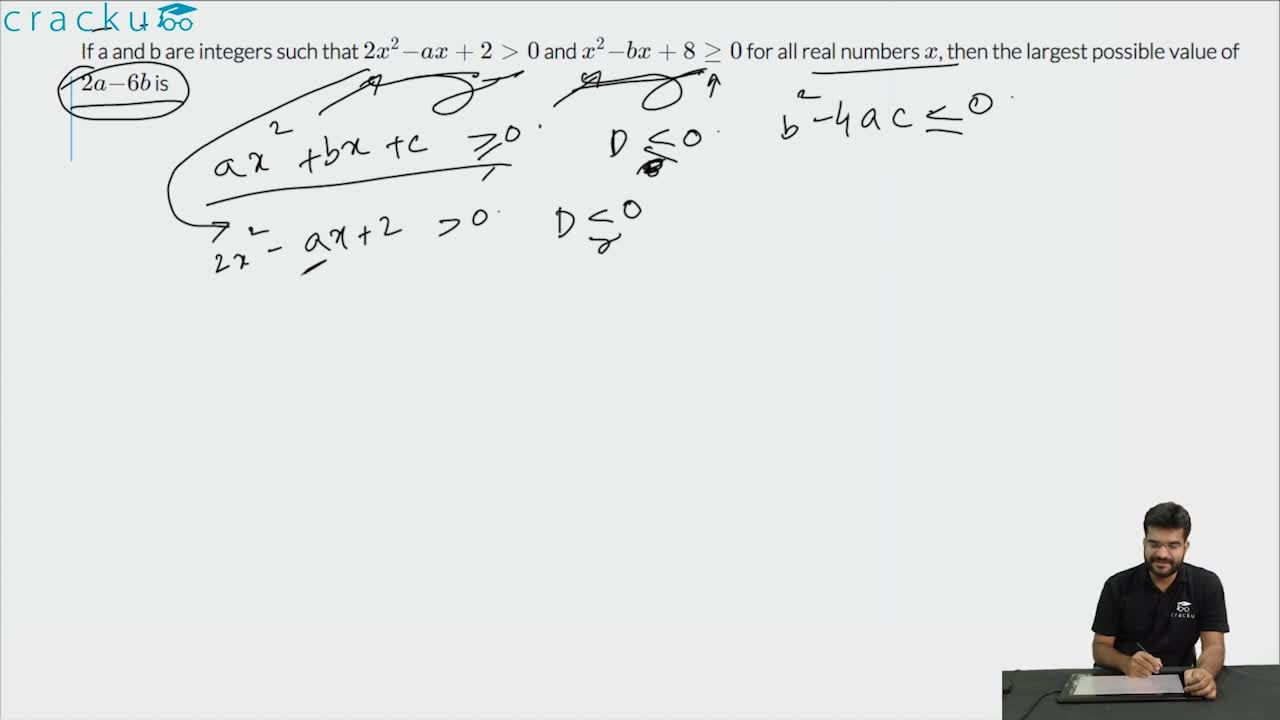Question 26

# If a and b are integers such that $$2x^2−ax+2>0$$ and $$x^2−bx+8≥0$$ for all real numbers $$x$$, then the largest possible value of $$2a−6b$$ is

Solution

Let f(x) = $$2x^2−ax+2$$. We can see that f(x) is a quadratic function.

For, f(x) > 0, Discriminant (D) < 0

$$\Rightarrow$$ $$(-a)^2-4*2*2<0$$

$$\Rightarrow$$ (a-4)(a+4)<0

$$\Rightarrow$$ a $$\epsilon$$ (-4, 4)

Therefore, integer values that 'a' can take = {-3, -2, -1, 0, 1, 2, 3}

Let g(x) = $$x^2−bx+8$$. We can see that g(x) is also a quadratic function.

For, g(x)≥0, Discriminant (D) $$\leq$$ 0

$$\Rightarrow$$ $$(-b)^2-4*8*1<0$$

$$\Rightarrow$$ $$(b-\sqrt{32})(b+\sqrt{32})<0$$

$$\Rightarrow$$ b $$\epsilon$$ (-$$\sqrt{32}$$, $$\sqrt{32}$$)

Therefore, integer values that 'b' can take = {-5, -4, -3, -2, -1, 0, 1, 2, 3, 4, 5}

We have to find out the largest possible value of $$2a−6b$$. The largest possible value will occur when 'a' is maximum and 'b' is minimum.

a$$_{max}$$ = 3, b$$_{min}$$ = -5

Therefore, the largest possible value of $$2a−6b$$ = 2*3 - 6*(-5) = 36.

### View Video Solution##### Pavan Sureja

4 years ago

why D<0 for 1st f(x) ??

##### Varun Tawde

4 years ago

f(x) > 0, Discriminant (D) < 0 why so??